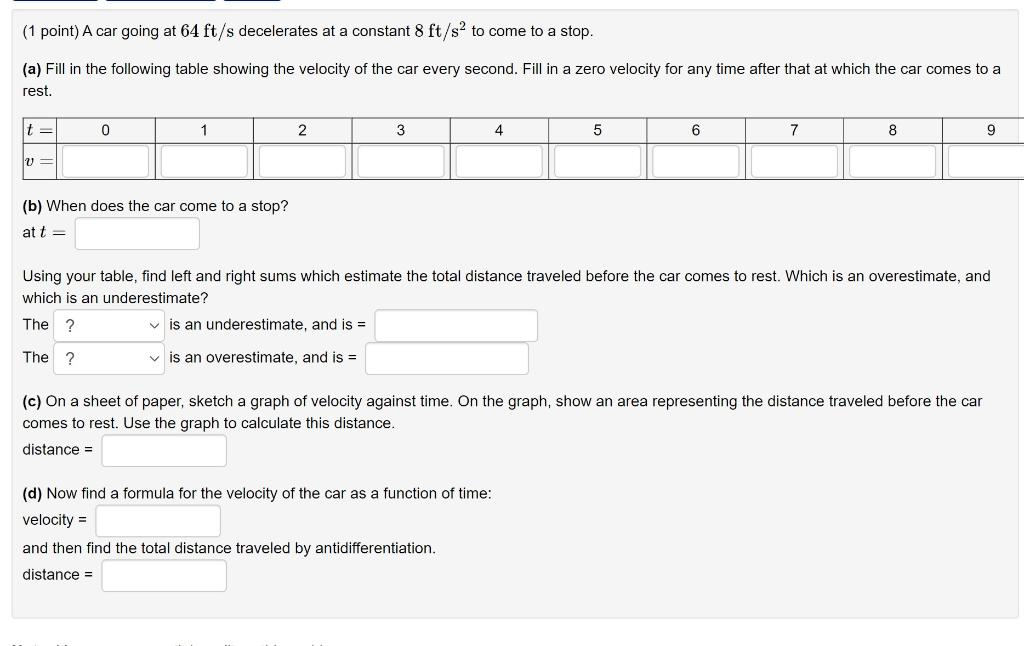# Question Solved1 Answer(1 point) A car going at 64 ft/s decelerates at a constant 8 ft/sº to come to a stop. (a) Fill in the following table showing the velocity of the car every second. Fill in a zero velocity for any time after that at which the car comes to a rest. t = 0 1 2 3 4 5 6 7 8 9 = (b) When does the car come to a stop? at t = Using your table, find left and right sums which estimate the total distance traveled before the car comes to rest. Which is an overestimate, and which is an underestimate? The ? is an underestimate, and is = The ? is an overestimate, and is = (c) On a sheet of paper, sketch a graph of velocity against time. On the graph, show an area representing the distance traveled before the car comes to rest. Use the graph to calculate this distance. distance = (d) Now find a formula for the velocity of the car as a function of time: velocity = and then find the total distance traveled by antidifferentiation. distance =Transcribed Image Text: (1 point) A car going at 64 ft/s decelerates at a constant 8 ft/sº to come to a stop. (a) Fill in the following table showing the velocity of the car every second. Fill in a zero velocity for any time after that at which the car comes to a rest. t = 0 1 2 3 4 5 6 7 8 9 = (b) When does the car come to a stop? at t = Using your table, find left and right sums which estimate the total distance traveled before the car comes to rest. Which is an overestimate, and which is an underestimate? The ? is an underestimate, and is = The ? is an overestimate, and is = (c) On a sheet of paper, sketch a graph of velocity against time. On the graph, show an area representing the distance traveled before the car comes to rest. Use the graph to calculate this distance. distance = (d) Now find a formula for the velocity of the car as a function of time: velocity = and then find the total distance traveled by antidifferentiation. distance =
More
Transcribed Image Text: (1 point) A car going at 64 ft/s decelerates at a constant 8 ft/sº to come to a stop. (a) Fill in the following table showing the velocity of the car every second. Fill in a zero velocity for any time after that at which the car comes to a rest. t = 0 1 2 3 4 5 6 7 8 9 = (b) When does the car come to a stop? at t = Using your table, find left and right sums which estimate the total distance traveled before the car comes to rest. Which is an overestimate, and which is an underestimate? The ? is an underestimate, and is = The ? is an overestimate, and is = (c) On a sheet of paper, sketch a graph of velocity against time. On the graph, show an area representing the distance traveled before the car comes to rest. Use the graph to calculate this distance. distance = (d) Now find a formula for the velocity of the car as a function of time: velocity = and then find the total distance traveled by antidifferentiation. distance =